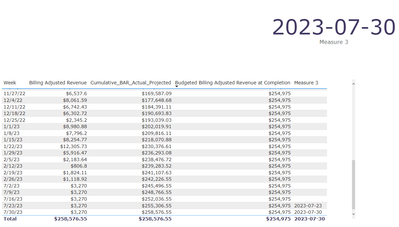cancel
Showing results for
Did you mean:Helper III

## Creating a measure to return week if one column is greater than another between two unrelated tables

Hi Everyone,
I've been trying to create a measure which will return the week when one column (Cumulative_BAR_Actual_Projected) is greater than another column (Budgeted Billing Adjusted Revenue at Completion). The catch is that these two columns are from two unrelated tables. I have a table visual like the one below.The first 3 columns come from one table and the last column, "Budgeted Billing Adjusted Revenue at Completion" comes from another table. I need to create a measure which will return the week when "Cumulative_BAR_Actual_Projected" is greater than "Budgeted Billing Adjusted Revenue at Completion". I can add it as a column to the table visual and shwo the latest week in the card visual at the bottom. I have tried a few different measure but they don't seem to work. Here are some measures I tried:

Exceeded_Budget_Week2 =
VAR TotalRevenue = CALCULATE(SUM('FACT_Ops_Data_by_Wk'[Billing Adjusted Revenue]))
VAR BudgetedRevenue = SUM('FACT_Portfolio_Perf'[Budgeted Billing Adjusted Revenue at Completion])
RETURN
MINX(
FILTER(
ALLSELECTED('FACT_Ops_Data_by_Wk'[Week]),
CALCULATE(sum('FACT_Ops_Data_by_Wk'[Billing Adjusted Revenue]), ALLEXCEPT('FACT_Ops_Data_by_Wk', 'FACT_Ops_Data_by_Wk'[Week])) > BudgetedRevenue
),
'FACT_Ops_Data_by_Wk'[Week]
)

First Week Exceeding Budgeted BAR = CALCULATE( MAX('Table1'[Week]), FILTER( ALL('Table1'), 'Table1'[Cumulative_BAR_Actual_Projected] > MAX('Table2'[Budgeted Billing Adjusted Revenue at Completion]) ) )

Any help is appreciated. Thank you!

2 ACCEPTED SOLUTIONSCommunity Support

Hi @akkitek ,

``````Measure 3 = VAR A=ADDCOLUMNS(ALLSELECTED(FACT_Ops_Data_by_Wk[Week]),"tEST1",[Cumulative_BAR_Actual_Projected],"TEST2",SUM(FACT_Portfolio_Perf[Budgeted Billing Adjusted Revenue at Completion]),"TEST4",SUM(FACT_Ops_Data_by_Wk[Billing Adjusted Revenue]))
RETURN MAXX(FILTER(B,[Week] IN VALUES(FACT_Ops_Data_by_Wk[Week])),[TEST3])``````Best Regards,

Neeko Tang

If this post  helps, then please consider Accept it as the solution  to help the other members find it more quickly.Helper III

I also found another way to do this.
I first created a column, then created a measure to achieve this:

``````BBAR per Project =
SUMX(
FILTER(
FACT_Ops_Data_by_Wk,
FACT_Ops_Data_by_Wk[Project Code] = EARLIER(FACT_Ops_Data_by_Wk[Project Code])
),
)``````

``````Week exceeding =
IF(
[Cumulative_BAR_Actual_Projected] > SELECTEDVALUE(FACT_Ops_Data_by_Wk[BBAR per Project]), SELECTEDVALUE(FACT_Ops_Data_by_Wk[Week]),BLANK())``````

3 REPLIES 3Helper III

I also found another way to do this.
I first created a column, then created a measure to achieve this:

``````BBAR per Project =
SUMX(
FILTER(
FACT_Ops_Data_by_Wk,
FACT_Ops_Data_by_Wk[Project Code] = EARLIER(FACT_Ops_Data_by_Wk[Project Code])
),
)``````

``````Week exceeding =
IF(
[Cumulative_BAR_Actual_Projected] > SELECTEDVALUE(FACT_Ops_Data_by_Wk[BBAR per Project]), SELECTEDVALUE(FACT_Ops_Data_by_Wk[Week]),BLANK())``````Community Support

Hi @akkitek ,

``````Measure 3 = VAR A=ADDCOLUMNS(ALLSELECTED(FACT_Ops_Data_by_Wk[Week]),"tEST1",[Cumulative_BAR_Actual_Projected],"TEST2",SUM(FACT_Portfolio_Perf[Budgeted Billing Adjusted Revenue at Completion]),"TEST4",SUM(FACT_Ops_Data_by_Wk[Billing Adjusted Revenue]))
RETURN MAXX(FILTER(B,[Week] IN VALUES(FACT_Ops_Data_by_Wk[Week])),[TEST3])``````Best Regards,

Neeko Tang

If this post  helps, then please consider Accept it as the solution  to help the other members find it more quickly.Helper III

Thanks @v-tangjie-msft ! This worked perfectly!Announcements#### Exclusive opportunity for Women!

Join us for a free, hands-on Microsoft workshop led by women trainers for women where you will learn how to build a Dashboard in a Day!#### Power Platform Conference-Power BI and Fabric Sessions

Join us Oct 1 - 6 in Las Vegas for the Microsoft Power Platform Conference.Top Solution Authors
Top Kudoed Authors
Users online (2,826)